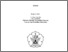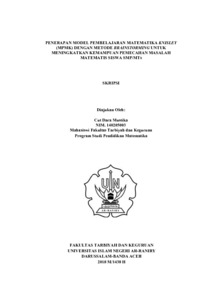# Penerapan Model Pembelajaran Matematika Knisley (MPMK) Dengan Metode Brainstorming Untuk Meningkatkan Kemampuan Pemecahan Masalah Matematis Siswa SMP/MTs

Cut Dara Mustika, 140205003 (2019) Penerapan Model Pembelajaran Matematika Knisley (MPMK) Dengan Metode Brainstorming Untuk Meningkatkan Kemampuan Pemecahan Masalah Matematis Siswa SMP/MTs. Skripsi thesis, UIN Ar-Raniry Banda Aceh.Preview
Text (Penerapan Model Pembelajaran Matematika Knisley (MPMK) Dengan Metode Brainstorming Untuk Meningkatkan Kemampuan Pemecahan Masalah Matematis Siswa SMP/MTs)
SKRIPSI FULL.pdf - Published Version
Available under License Creative Commons Attribution.

Download (3MB) | Preview

## Abstract

According to the NCTM, the ability of problem solving is one of the important capabilities which should be possessed by the students to solve various problems such as mathematical problems and those related to their daily lives. One of the learning models which is assumed to be applied to improve the ability of mathematical problem solving is Knisley mathematical learning model (MPMK) through brainstorming method. The purpose of this research is to find out the improvement of problem solving ability after knisley mathematical learning model (MPMK) through brainstorming method has been applied and to figure out the comparison of student problem solving ability applied by using Knisley mathematical learning model (MPMK) through brainstorming method. the student problem solving ability is applied using conventional learning method on the student od Junior high school SMP/MTs. The research methodology used is quasi-experiment with design of control group pretest-posttest design. The research sample consists of 33 students of experimental class and 32 students of control class. The sampling technique of this study is simple random sampling. The data are collected using student problem solving ability test. (1) the description of student problem solving ability is based on the indicators viz: 1)the ability to write elements previously recognized as 3% increased up to 97%; 2) to write the elements which are questioned from 5% becoming 95%; 3) constructing mathematical model from 8% becoming 92%; 4) applying procedure (computational operation) which is previously known as 6% becoming 94%; 5) rechecking the result of problem solving completion which is preciously known as 11% increasing up to 89%. 2) based on the independent t-experiment, it is found that t compute=7,60 and t table = 1,67, or 7,60>1,67 therefore H 0 is rejected. Based on the finding, it is concluded that the student mathematical problem solving ability after Knisley mathematical learning model has been applied through brainstorming method is better compared to the conventional learning model.

Keywords : Knisley mathematical learning model (MPMK), brainstorming method, mathematical problem solving

Item Type: Thesis (Skripsi) Pembimbing 1. Dr.M.Duskri, M.Kes. 2. Novi Trina Sari, M.Pd Model Pembelajaran Matematika Knisley (MPMK), Metode Brainstorming, Kemampuan Pemecahan Masalah 500 Sciences (Ilmu Pengetahuan Alam dan Matematika)500 Sciences (Ilmu Pengetahuan Alam dan Matematika) > 510 Mathematics (Matematika)500 Sciences (Ilmu Pengetahuan Alam dan Matematika) > 510 Mathematics (Matematika) > 512 Aljabar > 512.9 Dasar-dasar Aljabar Fakultas Tarbiyah dan Keguruan > S1 Pendidikan Matematika Cut Dara Mustika cut 24 Apr 2019 07:41 24 Apr 2019 07:41 https://repository.ar-raniry.ac.id/id/eprint/7292

### Actions (login required)View Item
TOP